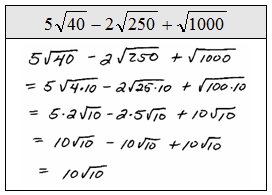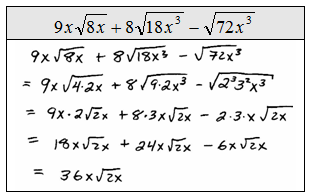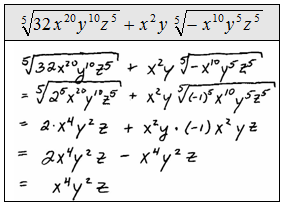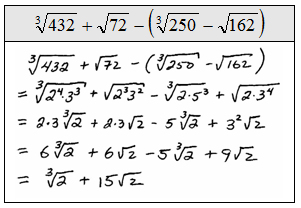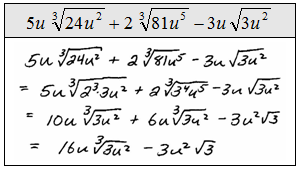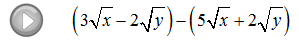## Algebra

Showing posts with label prime factor. Show all posts
Showing posts with label prime factor. Show all posts

To add or subtract radical expressions, simplify first and then add like terms if there are any.  The radical parts of the terms must be exactly the same before we can add them. (Assume all variables are positive.)

Perform the operations.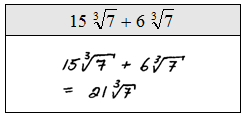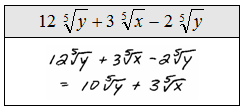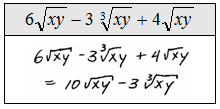Be sure to follow the correct order of operations.

Simplify.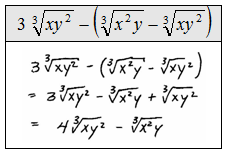Unsimplified rational expressions may look as if they have no like terms, but first try simplifying and then check for like terms.Simplifying is a challenge for many students. In particular, the numerical part is the source of confusion.  It is much easier to deal with the prime factors of the number in a radical than it is with the number itself. Create a factor tree and determine the prime factorization of all numbers before simplifying radical expressions.

Simplify:Begin by determining the prime factorizations of 108 and 864.Substitute the prime factorization in and then simplify.Simplify.